The Dodecagons ClipArt gallery contains 10 illustrations of geometric shapes that have 12 sides. This most often refers to the regular dodecagons, those that have 12 equal length sides and 12 angles that each equal 150 degrees.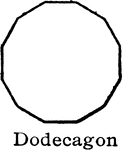### Dodecagon

"A polygon of...twelve sides is [a] dodecagon." —Hallock 1905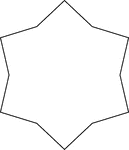### Concave Equilateral Dodecagon

A 6-point star made from a non-regular concave dodecagon in which all sides are equal in length. There…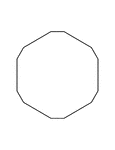### Irregular Convex Dodecagon

Illustration of an irregular convex dodecagon. This polygon has some symmetry.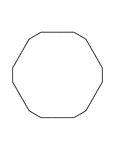### Irregular Convex Dodecagon

Illustration of an irregular convex dodecagon. This polygon has some symmetry.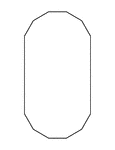### Irregular Convex Dodecagon

Illustration of an irregular convex dodecagon. This polygon has some symmetry.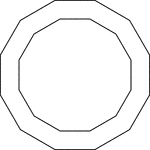### 2 Concentric Dodecagons

Illustration of 2 regular concentric dodecagons.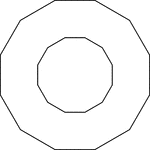### 2 Concentric Dodecagons

Illustration of 2 regular concentric dodecagons.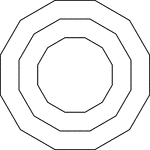### 3 Concentric Dodecagons

Illustration of 3 regular concentric dodecagons that are equally spaced.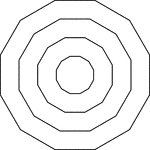### 4 Concentric Dodecagons

Illustration of 4 regular concentric dodecagons that are equally spaced.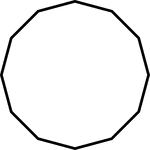### 12-sided Polygon

Polygon consisting of 12 sides